# Dynamic Resource Scheduler for Distributed Deep Learning Training on Kubeflow

Distributed deep learning is a method of machine learning that is used today due to its many advantages. One of the many tools used to train distributed deep learning model is Kubeflow, which runs on top of Kubernetes. Kubeflow provides utility and workflows to ease the process of managing, training, and deploying machine learning models. Kubeflow use tf-operator as a custom controller to manage job scheduling. I tried to improve the scheduling capability of Kubeflow by developing a custom controller inspired by DRAGON and OASIS to replace the existing tf-operator.

# Basic Introduction

## Distributed Machine Learning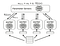Distributed machine learning, from DRAGON: A Dynamic Scheduling and Scaling Controller for Managing Distributed Deep Learning Jobs in Kubernetes Cluster

# Dynamic Scheduler Basic Ideas

1. Gang Scheduling
2. Autoscaling
3. Priority Queueing

## Gang Scheduling

Gang scheduling is a scheduling methodology that group all workers to a single node. It will minimize the amount of communication overhead needed by each job, hence increasing the overall training time.

# Implementation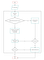Scheduler Architecture

## Weighting Algorithm

`INPUT:  Job queue Q, job JOUTPUT:  Job queue Q with job J 1:  if len(Q) == 0 do 2:    Q.append(J) 3:    return Q 4:  end if 5:  Initialize R = J CPU requirement + J Memory requirement 6:  Initialize U = J Utility 7:    for q in Q do 8:      Initialize r = q CPU requirement + q Memory requirement 9:      Initialize u = q Utility 10:     if (U > u) or (U == u and R > r) do 11:       Initialize F = queue of job so that U > u 12:       Initialize f = queue of job so that U <= u 13:       Q.append(F) 14:       Q.append(J) 15:       Q.append(f) 16:       return Q 17:     end if 18:   end for19: Q.append(J) 20: return Q`

## Gang Scheduling

`INPUT:  Pod requests PR, Node Resources NR 1:  for n in NR do 2:    Initialize stop = False 3:    Initialize oneNodeOk = True 4:    for r in PR do 5:      if (n free CPU < r required CPU) or (n free 6:      Memory < r required Memory) do 7:        oneNodeOk = False 8:        stop = True 9:        n free CPU -= r required CPU 10:       n free Memory -= r required Memory11:     end if11:   end for 12:   if stop do break 13: end for 14: if oneNodeOk do schedule all r in one node 15: else do schedule all r in the first available node`

## Scale-down

1. The available resource can satisfy the high priority job → success scale-down.
2. The number of worker of every job with lower priority has been reduced its minimum value → failed scale-down.
`INPUT:  High priority job J, running queue Q, node resources NR OUTPUT:  Success S1:  for q in Q do 2:    Initialize i = 0 3:    Initialize maxDeleteCount = q current replica count – 4:    q minimum replica count 5:    Initialize stop = False 6:    for n in NR do 7:      if J can be scheduled in n do 8:        Initialize S = True 9:        return S 10:     end if 11:     if i >= maxDeleteCount do 12:       Initialize stop = True 13:       break 14:     end if 15:     Initialize res = node resources of n 16:     res free CPU += q required CPU 17:     res free Memory += q required Memory 18:     i++ 19:     if stop do break 20:   end for 21: end for 22: if J can be scheduled in n do 23:   Initialize S = True 24:   return S 25: end if 26: return S`

## Scale-up

`INPUT:  Running queue Q, node resources NR OUTPUT:  Success S1:  Initialize i = 0 2:  Initialize S = False 3:  for n in NR do 4:    for q in Q do 5:      Initialize maxScaleUpNum = q maximum replica count 6:      - q current replica count 7:      Initialize r = q replica request for worker 8:      for j -> maxScaleUpNum do 9:        if (n free CPU < r required CPU) or (n free 10:       Memory < r required Memory) do 11:         Initialize stop = True 12:         break 13:       end if14:     end for 15:     n free CPU -= r required CPU 16:     n free Memory -= r required Memory 17:     initialize one new worker in cluster 18:     Initialize S = True 19:   end for 20:   if stop do break 21: if S do break 22: return S`

## Scheduling Algorithm

`INPUT:  Waiting queue W, running queue R, node resources NR 1:  Initialize scaleDownFlag = False 2:  if len(W) > 0 do 3:    Initialize U = max waiting job utility 4:    Initialize u = max running job utility 5:    if U > u do 6:      Initialize h = first job in W 7:      if h can be scheduled do 8:        W.remove(h) 9:        R.add(h) 10:     end if 11:     run ScaleDown function 12:     if ScaleDown is successful do 13:       Initialize scaleDownFlag = True 14:     end if 15:   end if 16: end if 17: if h == nil or scaleDownFlag do 18:   for w in W do 19:     if w can be scheduled do 20:       W.remove(w) 21:       R.add(w) 22:       return 23:     end if 24:   end for 25: end if 26: run ScaleUp function`

# Evaluation

## Specifications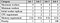Specifications for experiment 1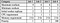Specifications for experiment 2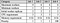Specifications for experiment 3

## Result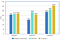Evaluation result for experiment 1, 2, and 3. Lower value is better.

# Conclusion

An up and coming software engineer, learning to love to write.

## More from Fadhriga Bestari

An up and coming software engineer, learning to love to write.